#### Simulation GAMES• Simulation
• Management
• Strategy• Action
• Simulation
• Shooter
• Building
• Horror• Nudity
• Simulation
• Casual
• VR• Action
• Nudity
• Simulation
• Mature• Casual
• Simulation
• Action
• Survival
• Visual Novel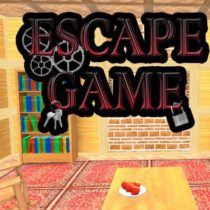• Casual
• Puzzle
• Point & Click
• Hidden Object
• Strategy
• Simulation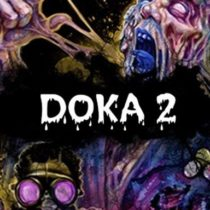• Action
• Simulation
• RPG
• Casual
• Survival
• Mature
• Nudity• Strategy
• Casual
• Simulation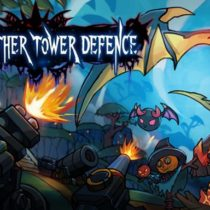• Strategy
• Casual
• Tower Defense
• Simulation• Casual
• Simulation
• Visual Novel
• Nudity
• Anime
• Mature• Simulation
• Strategy
• Management
• Building• Simulation
• Casual
• Open World
• Building
• Action
• Strategy
• VR• Visual Novel
• Nudity
• Anime
• Casual
• Simulation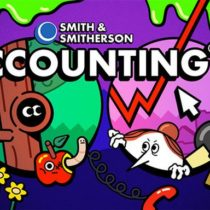• Action
• VR
• Casual
• Simulation
• Horror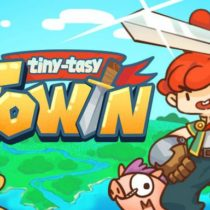• Casual
• Simulation
• RPG• Simulation
• Strategy
• Nudity
• Horror